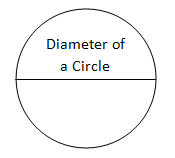# 14 March 2018 is celebrated as PI (π) day globally

PI (π) is celebrated globally on 14th March 2018. Let us celebrate the pie (pi) day with a little bite of pie. PI day was celebrated as National PI day in America with pie eating and having discussions about PI. Travel with us to celebrate PI Day with crystal clear details. Archimedes the ancient Greek scientist was the first to calculate the accurate PI value. William Jones, a mathematician coined the word PI, is the first letter in the Greek word perimitros, which means “perimeter. The PI is used to define the ratio of the circle’s circumference to its diameter.

We all have a doubt on why we use PI and why do we celebrate PI Day?The circumference of the circle is 3 times of its diameter and .14159. This was clearly stated by Archimedes, he used the polygons with many sides to approximate circles to find the solution. PI is an irrational number. The value of PI is 3.1415. PI is used in Physics and mathematics as to solve important equations. Value of PI is a never ending number, any number divides PI, infinity will be answer. So far we have calculated the value of PI to ten trillion digits and the numbers in the answer has never repeated.

We IBPS Guide wish you the special PI day, educate the people around you the importance of PI(π) to everyone.